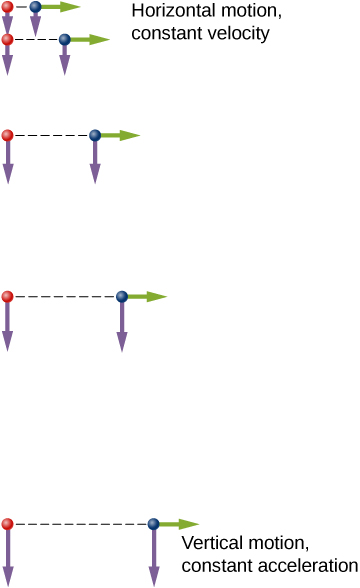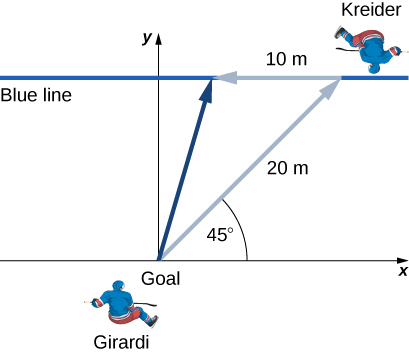# 4.1 Displacement and velocity vectors  (Page 4/7)

 Page 4 / 7

An example illustrating the independence of vertical and horizontal motions is given by two baseballs. One baseball is dropped from rest. At the same instant, another is thrown horizontally from the same height and it follows a curved path. A stroboscope captures the positions of the balls at fixed time intervals as they fall ( [link] ).A diagram of the motions of two identical balls: one falls from rest and the other has an initial horizontal velocity. Each subsequent position is an equal time interval. Arrows represent the horizontal and vertical velocities at each position. The ball on the right has an initial horizontal velocity whereas the ball on the left has no horizontal velocity. Despite the difference in horizontal velocities, the vertical velocities and positions are identical for both balls, which shows the vertical and horizontal motions are independent.

It is remarkable that for each flash of the strobe, the vertical positions of the two balls are the same. This similarity implies vertical motion is independent of whether the ball is moving horizontally. (Assuming no air resistance, the vertical motion of a falling object is influenced by gravity only, not by any horizontal forces.) Careful examination of the ball thrown horizontally shows it travels the same horizontal distance between flashes. This is because there are no additional forces on the ball in the horizontal direction after it is thrown. This result means horizontal velocity is constant and is affected neither by vertical motion nor by gravity (which is vertical). Note this case is true for ideal conditions only. In the real world, air resistance affects the speed of the balls in both directions.

The two-dimensional curved path of the horizontally thrown ball is composed of two independent one-dimensional motions (horizontal and vertical). The key to analyzing such motion, called projectile motion , is to resolve it into motions along perpendicular directions. Resolving two-dimensional motion into perpendicular components is possible because the components are independent.

## Summary

• The position function $\stackrel{\to }{r}\left(t\right)$ gives the position as a function of time of a particle moving in two or three dimensions. Graphically, it is a vector from the origin of a chosen coordinate system to the point where the particle is located at a specific time.
• The displacement vector $\text{Δ}\stackrel{\to }{r}$ gives the shortest distance between any two points on the trajectory of a particle in two or three dimensions.
• Instantaneous velocity gives the speed and direction of a particle at a specific time on its trajectory in two or three dimensions, and is a vector in two and three dimensions.
• The velocity vector is tangent to the trajectory of the particle.
• Displacement $\stackrel{\to }{r}\left(t\right)$ can be written as a vector sum of the one-dimensional displacements $\stackrel{\to }{x}\left(t\right),\stackrel{\to }{y}\left(t\right),\stackrel{\to }{z}\left(t\right)$ along the x , y , and z directions.
• Velocity $\stackrel{\to }{v}\left(t\right)$ can be written as a vector sum of the one-dimensional velocities ${v}_{x}\left(t\right),{v}_{y}\left(t\right),{v}_{z}\left(t\right)$ along the x , y , and z directions.
• Motion in any given direction is independent of motion in a perpendicular direction.

## Conceptual questions

What form does the trajectory of a particle have if the distance from any point A to point B is equal to the magnitude of the displacement from A to B ?

straight line

Give an example of a trajectory in two or three dimensions caused by independent perpendicular motions.

If the instantaneous velocity is zero, what can be said about the slope of the position function?

The slope must be zero because the velocity vector is tangent to the graph of the position function.

## Problems

The coordinates of a particle in a rectangular coordinate system are (1.0, –4.0, 6.0). What is the position vector of the particle?

$\stackrel{\to }{r}=1.0\stackrel{^}{i}-4.0\stackrel{^}{j}+6.0\stackrel{^}{k}$

The position of a particle changes from ${\stackrel{\to }{r}}_{1}=\left(2.0\text{​}\stackrel{^}{i}+3.0\stackrel{^}{j}\right)\text{cm}$ to ${\stackrel{\to }{r}}_{2}=\left(-4.0\stackrel{^}{i}+3.0\stackrel{^}{j}\right)\phantom{\rule{0.2em}{0ex}}\text{cm}.$ What is the particle’s displacement?

The 18th hole at Pebble Beach Golf Course is a dogleg to the left of length 496.0 m. The fairway off the tee is taken to be the x direction. A golfer hits his tee shot a distance of 300.0 m, corresponding to a displacement $\text{Δ}{\stackrel{\to }{r}}_{1}=300.0\phantom{\rule{0.2em}{0ex}}\text{m}\stackrel{^}{i},$ and hits his second shot 189.0 m with a displacement $\text{Δ}{\stackrel{\to }{r}}_{2}=172.0\phantom{\rule{0.2em}{0ex}}\text{m}\stackrel{^}{i}+80.3\phantom{\rule{0.2em}{0ex}}\text{m}\stackrel{^}{j}.$ What is the final displacement of the golf ball from the tee?

$\text{Δ}{\stackrel{\to }{r}}_{\text{Total}}=472.0\phantom{\rule{0.2em}{0ex}}\text{m}\stackrel{^}{i}+80.3\phantom{\rule{0.2em}{0ex}}\text{m}\stackrel{^}{j}$

A bird flies straight northeast a distance of 95.0 km for 3.0 h. With the x -axis due east and the y -axis due north, what is the displacement in unit vector notation for the bird? What is the average velocity for the trip?

A cyclist rides 5.0 km due east, then 10.0 km $20\text{°}$ west of north. From this point she rides 8.0 km due west. What is the final displacement from where the cyclist started?

$\text{Sum of displacements}=-6.4\phantom{\rule{0.2em}{0ex}}\text{km}\stackrel{^}{i}+9.4\phantom{\rule{0.2em}{0ex}}\text{km}\stackrel{^}{j}$

New York Rangers defenseman Daniel Girardi stands at the goal and passes a hockey puck 20 m and $45\text{°}$ from straight down the ice to left wing Chris Kreider waiting at the blue line. Kreider waits for Girardi to reach the blue line and passes the puck directly across the ice to him 10 m away. What is the final displacement of the puck? See the following figure.The position of a particle is $\stackrel{\to }{r}\left(t\right)=4.0{t}^{2}\stackrel{^}{i}-3.0\stackrel{^}{j}+2.0{t}^{3}\stackrel{^}{k}\text{m}.$ (a) What is the velocity of the particle at 0 s and at $1.0$ s? (b) What is the average velocity between 0 s and $1.0$ s?

a. $\stackrel{\to }{v}\left(t\right)=8.0t\stackrel{^}{i}+6.0{t}^{2}\stackrel{^}{k},\phantom{\rule{0.7em}{0ex}}\stackrel{\to }{v}\left(0\right)=0,\phantom{\rule{0.7em}{0ex}}\stackrel{\to }{v}\left(1.0\right)=8.0\stackrel{^}{i}+6.0\stackrel{^}{k}\text{m/s}$ ,
b. ${\stackrel{\to }{v}}_{\text{avg}}=4.0\text{​}\stackrel{^}{i}+2.0\stackrel{^}{k}\phantom{\rule{0.2em}{0ex}}\text{m/s}$

Clay Matthews, a linebacker for the Green Bay Packers, can reach a speed of 10.0 m/s. At the start of a play, Matthews runs downfield at $45\text{°}$ with respect to the 50-yard line and covers 8.0 m in 1 s. He then runs straight down the field at $90\text{°}$ with respect to the 50-yard line for 12 m, with an elapsed time of 1.2 s. (a) What is Matthews’ final displacement from the start of the play? (b) What is his average velocity?

The F-35B Lighting II is a short-takeoff and vertical landing fighter jet. If it does a vertical takeoff to 20.00-m height above the ground and then follows a flight path angled at $30\text{°}$ with respect to the ground for 20.00 km, what is the final displacement?

$\text{Δ}{\stackrel{\to }{r}}_{1}=20.00\phantom{\rule{0.2em}{0ex}}\text{m}\stackrel{^}{j},\text{Δ}{\stackrel{\to }{r}}_{2}=\left(2.000\phantom{\rule{0.2em}{0ex}}×\phantom{\rule{0.2em}{0ex}}{10}^{4}\text{m}\right)\phantom{\rule{0.2em}{0ex}}\left(\text{cos}30\text{°}\stackrel{^}{i}+\text{sin}\phantom{\rule{0.2em}{0ex}}30\text{°}\stackrel{^}{j}\right)$
$\text{Δ}\stackrel{\to }{r}=1.700\phantom{\rule{0.2em}{0ex}}×\phantom{\rule{0.2em}{0ex}}{10}^{4}\text{m}\stackrel{^}{i}+1.002\phantom{\rule{0.2em}{0ex}}×\phantom{\rule{0.2em}{0ex}}{10}^{4}\text{m}\stackrel{^}{j}$

what is a wave?
show that coefficient of friction of solid block inclined at an angle is equivalent to trignometric tangent of angle
DAVID
thanks for that definition.
Hi everyone please can dere be motion without force?
Lafon
no...
Enyia
Thanks
Lafon
hi
Omomaro
whats is schrodinger equation
Omomaro
l went spiral spring
Xalat
what is position?
position is simply where you are or where you were
Shii
position is the location of an object with respect to a two or three dimensional axes or space.
Bamidele
Can dere be motion without force?
Lafon
what is the law of homogeinity?
two electric lines of force never interested each other. why?
proof that for BBC lattice structure 4r\root 5 and find Apf for the BBC structure
what is physics?
physics is deine as the specific measrument of of volume, area,nd distances...
Olakojo
if a string of 2m is suspended an an extended 3m elasticity is been applied.... is hooks law obeyed?
Enyia
if a string of 2m is suspended an an extended 3m elasticity is been applied.... is hooks law obeyed?
Enyia
yes
Alex
proof that for a BBC lattice structure a= 4r/ root 5 find the APF for the BBC structure
Eric
if a string of 2m is suspended an an extended 3m elasticity is been applied.... is hooks law obeyed?
tell me conceptual quetions of mechanics
I want to solve a physical question
ahmed
ok
PUBG
a displacement vector has a magnitude of 1.62km and point due north . another displacement vector B has a magnitude of 2.48 km and points due east.determine the magnitude and direction of (a) a+ b and (b) a_ b
quantum
George
a+b=2.9
SUNJO
a+b
Yekeen
use Pythogorous
Dhritwan
A student opens a 12kgs door by applying a constant force of 40N at a perpendicular distance of 0.9m from the hinges. if the door is 2.0m high and 1.0m wide determine the magnitude of the angular acceleration of the door. ( assume that the door rotates freely on its hinges.) please assist me to d
Mike
what is conditions met to produce shm
what is shm
Manzoor
shm?
Grant
Why is Maxwell saying that light is an electromagnetic wave?
Bong
1st condition; It(th e BBC's system) must have some inertia which will enable it to possess Kinetic energy 2. must be able to store potential energy
Calleb
I meant "the system" not the BBC'S....."
Calleb
Manzoor
kindly tell us the name of your university
Manzoor
GUlam Ishaq Khan INSTITUTE of engineering science
ali
Department of Environment Ionian University Zante Greece
why light wave travel faster than sounds
Why light travel faster than sounds?
ALI
Light travel faster than sound because it does not need any medium to travel through.
alhassan
when an aeroplane flies....why it does not fall on the earth?
Frazali
As an aeroplane moves, it hits a wind,we have the wind flowing at the upper and lower zone of the aeroplane, the one that is moving on the upper zone moves at a greater speed than that of the lower zone, this creates a low pressure on the upper zone and a greater pressure at the lower zone.
Kipkoech
which thing of aeroplane moves it upward?
Frazali
good question
Manzoor
Barataa
am pleased to join the group
Nesru
yea
caleb
It a privilege to be here
olajire
hi
Awode
hello
Manzoor
Light speed is more than sound speed. C=3×10*8m/s V=320-340 m/s
siva
A body of mass 2kg slides down a rough plane inclined to horizontal at 30degrees. find the energy that is wasted as a result of friction if the co-efficient of kinetic f
ten applications of Newton's second law of motion
Calculate the volume at S.T.P of a gas whose volume at -5° and 746 mmHgBy Lakeima RobertsBy Sebastian Sieczko...ByBy Jordon Humphreys